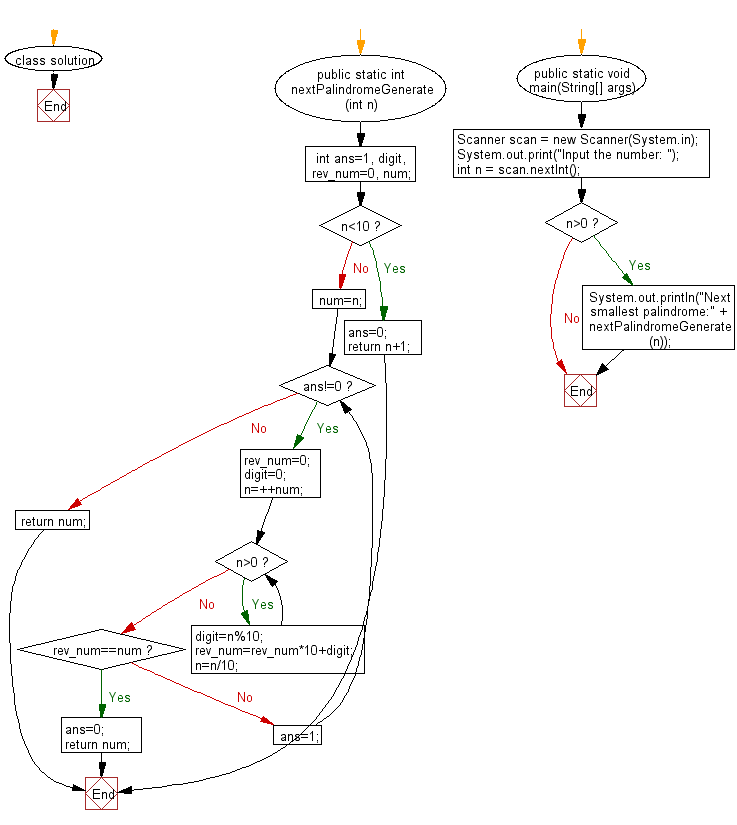﻿ Java Math Exercises: Find next smallest palindrome - w3resource# Java Math Exercises: Find next smallest palindrome

## Java Math Exercises: Exercise-22 with Solution

Write a Java program to find next smallest palindrome.

Sample Solution:

Java Code:

``````import java.util.*;

class solution {

public static int nextPalindromeGenerate(int n)
{
int ans=1, digit, rev_num=0, num;
//For single digit number, next smallest palindrome is n+1
if(n<10)
{
ans=0;
return n+1;
}

num=n;
while(ans!=0)
{   rev_num=0;digit=0;
n=++num;

while(n>0)      //rev_numersing the number
{
digit=n%10;
rev_num=rev_num*10+digit;
n=n/10;
}

if(rev_num==num)
{
ans=0;
return num;
}

else ans=1;
}
return num;
}

public static void main(String[] args)
{
Scanner scan = new Scanner(System.in);
System.out.print("Input the number: ");
int n = scan.nextInt();
if (n>0)
{
System.out.println("Next smallest palindrome:" + nextPalindromeGenerate(n));
}
}
}
``````

Sample Output:

```Input the number:  121
Next smallest palindrome:131
```

Flowchart:Java Code Editor:

What is the difficulty level of this exercise?

﻿

## Java: Tips of the Day

Parsing dates:

```import java.io.*;
import java.util.*;
import java.text.*;

String s = "2001/09/23 14:39";

SimpleDateFormat formatter = new SimpleDateFormat ("yyyy/MM/dd H:mm");
Date d = formatter.parse(s, new ParsePosition(0));
```